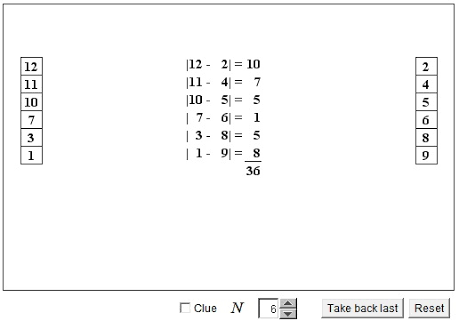# What Do You Know About Proizvolov's Identity?

10 Questions | Total Attempts: 104SettingsWhen it comes to mathematical expressions or equations, Proizvolov's identity is described as the identity that deals with the summation of the difference of the integers that are positive. The identity was discovered by Vyacheslav Proizvolov and has been used to solve numerous mathematical problems. We will like to test your knowledge about this theorem in this short quiz.

Related Topics
• 1.
When was the theorem discovered?
• A.

1976

• B.

1985

• C.

1987

• D.

1990

• 2.
Where was the theorem discovered?
• A.

• B.

1985 Olympic Games

• C.

Great Britain Theater

• D.

Soviet Union Square

• 3.
For Proizvolov's identity, what is S equal to?
• A.

N^2

• B.

N^3

• C.

2n

• D.

N-2

• 4.
When n=2, what is S equal to?
• A.

8

• B.

0

• C.

4

• D.

6

• 5.
When n=3, what is S equal to?
• A.

6

• B.

27

• C.

9

• D.

1

• 6.
When n = 4, what is S equal to?
• A.

16

• B.

8

• C.

2

• D.

64

• 7.
When n = 5, what is S equal to?
• A.

10

• B.

25

• C.

3

• D.

125

• 8.
When n = 9, what is S equal to?
• A.

81

• B.

18

• C.

7

• D.

27

• 9.
When n = 10, what is S equal to?
• A.

100

• B.

1000

• C.

8

• D.

20

• 10.
When n = 121, what is S equal to?
• A.

40.3

• B.

102

• C.

60.5

• D.

11